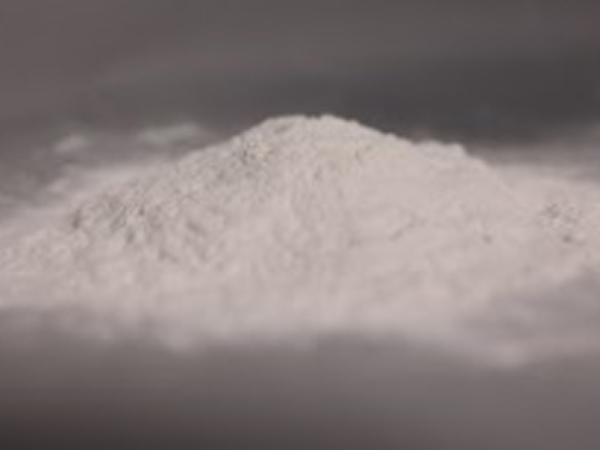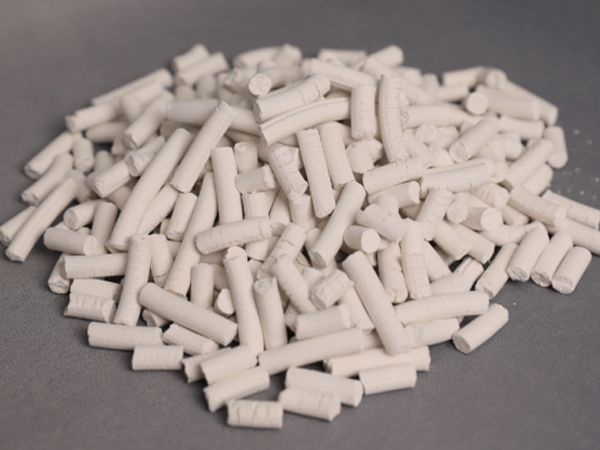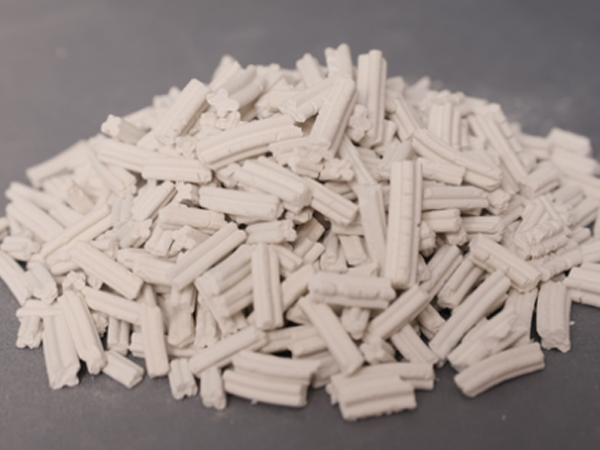# How to calculate the solubility of high specific surface calcium hydroxide

The solubility of calcium hydroxide, Ca(OH)2, increases with an increase in its surface area. This is because calcium hydroxide dissolves in water by a surface reaction, where water molecules interact with the calcium and hydroxide ions on the surface of the solid.

When the surface area of calcium hydroxide is increased, there is more surface area available for water molecules to interact with, which increases the rate and extent of dissolution. As a result, high surface area calcium hydroxide will dissolve more readily in water than low surface area calcium hydroxide.The solubility of calcium hydroxide in water is also affected by factors such as temperature and pH. At higher temperatures and higher pH values, the solubility of calcium hydroxide in water increases. However, these factors do not have as significant an effect on solubility as surface area.

The solubility of high specific surface calcium hydroxide can be calculated using the following equation:

Ksp = [Ca2+][OH-]^2

Where Ksp is the solubility product constant of calcium hydroxide, [Ca2+] is the concentration of calcium ions in solution, and [OH-] is the concentration of hydroxide ions in solution.

To calculate the solubility of calcium hydroxide, you need to know the value of Ksp and the concentrations of calcium and hydroxide ions in solution. The Ksp value for calcium hydroxide at room temperature (25°C) is approximately 5.5 x 10^-6.The concentration of calcium ions in solution can be calculated using the following equation:

[Ca2+] = (Ca(OH)2 dissolving in water) / (total volume of solution)

The concentration of hydroxide ions in solution can be calculated using the following equation:

[OH-] = 2 x [Ca2+]Once you have determined the concentrations of calcium and hydroxide ions in solution, you can plug them into the solubility product constant equation to calculate the solubility of calcium hydroxide.

It is important to note that the solubility of high specific surface calcium hydroxide may be different than that of regular calcium hydroxide due to its increased surface area. Therefore, experimental measurements may be necessary to determine the solubility of high specific surface calcium hydroxide.